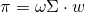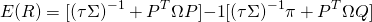# Black-Litterman Model

The Black-Litterman (BL) model is a model in finance proposed by Fischer Black and Robert Litterman. The model was developed in 1990 when both were working at Goldman Sachs. The model offers a simple way for managers to include ‘views’. In fact, the model is an extension of the mean-variance portfolio optimization approach of Markowitz. The resulting portfolio allocation will deviate from the market-capitalization weights.On this page, we discuss the Black-Litterman model assumptions, the Black-Litterman model formulas, and finally we explain how to extract market-implied returns from the market-capitalization weights. It is important to note that, to implement the model, matrix algebra is required. Thus, it is generally easier to implement the model using Python or MATLAB than by using Excel. Still, we include a Black-Litterman in Excel spreadsheet at the bottom of the page.

## Black-Litterman assumptions

The Black-Litterman model starts from market-implied expected returns as the natural starting point. Next, these market-implied expected returns are adjusted to reflect the views of the fund manager. The BL model makes two important assumptions:

• Asset returns are normally distributed
• The covariance matrix of asset returns is known

## Black-Litterman formulasWhere Sigma is the covariance matrix, omega is the risk-aversion parameter, and w is the vector with the market capitalization of the assets in the portfolio.

Next, let’s calculate the expected returns under the BL model. This is done by changing the market-implied returns using the views of the fund manager. First, we need to define a number of variables:

• P: matrix with the views. Each row is a view and each entry of the row represents the portfolios’ weights of each asset
• Omega: a diagonal covariance matrix with the uncertainty within each view
• Q: the expected return matrix of the portfolios from the views described in matrix P

Now we have all the ingredients to calculate expected returns## Black-Litterman versus Treynor-Black

It is important not to confuse the Black-Litterman model with the Treynor-Black model. For details on the latter model, see Treynor-Black model. This model is used to perform security analysis, whereas the Black-Litterman model is used for asset allocation.

## Summary

We discussed the basics behind the BL, a model that can be used to shrink expected returns such that they are consistent with market-implied returns. Despite the elegance of the model, it is not used that often.